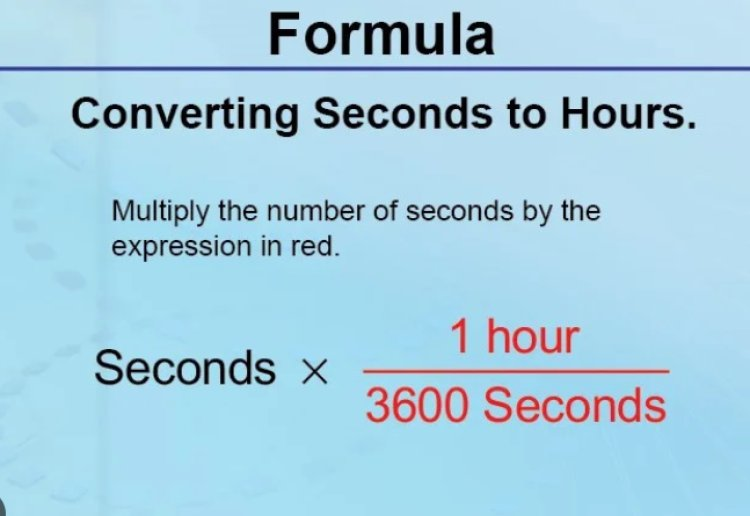# how to convert hour minutes to seconds in react native?

## In this tutorial, we will learn how to convert hour minutes to seconds in React Native. We will use two functions: one that converts hours and minutes to seconds and one that converts seconds to hours and minutes. The first function accepts two arguments, an integer representing the hours and an integer representing the minutes. It then multiplies the hours by 3600 and adds the minutes multiplied by 60 to get the total number of seconds.### Convert hours to minutes and seconds.

Time is an important element of our lives, and it's vital that we understand it. When converting hours to minutes and seconds, we must have a clear idea of the process. To convert hours to minutes, multiply the amount of hours by 60. For example, if two hours are converted to minutes, the result would be 120 minutes.

### Use this information to create a timer in React Native.

Set default time useState
const [defaulttime, setDefaultTime] = useState('02:25:45');
Call this function and get seconds to display
const getSeconds = e => {
var totalsec = 0;
if (e.length !== 0) {
var spl = e.split(':');
var hour = spl ? spl : 0;
var min = spl ? spl : 0;
var sec = spl ? spl : 0;
var newsec = spl ? sec.split('.') : 0;
var hs = hour * 3600;
var ms = min * 60;
if (newsec !== 0) {
totalsec = hs + ms + +newsec;
setSeconds(totalsec);
} else {
totalsec = hs + ms + +newsec;
setSeconds(totalsec);
}
}